# PSAT Math : Statistics

## Example Questions

1 2 13 14 15 16 17 18 19 21 Next →

### Example Question #49 : Probability

Set A: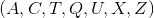Set B: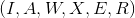One letter is drawn from Set A, and one from Set B. What is the probability of drawing a matching pair of letters?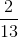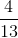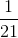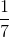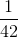Explanation:

Set A:Set B:Between Set A and Set B, there are two potential matching pairs of letters: AA and XX. The amount of possible combinations is the number of values in Set A, multiplied by the number of values in Set B,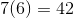.

Therefore, the probability of drawing a matching set is: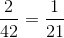### Example Question #61 : Discrete Probability

In a particular high school, 200 students are freshmen, 150 students are sophomores, 250 students are juniors, and 100 students are seniors. Twenty percent of freshmen are in honors classes, ten percent of sophomores are in honors classes, twelve percent of juniors are in honors classes, and thirty percent of seniors are in honors classes.

If a student is chosen at random, what is the probability that that student will be a student who attends honors classes?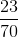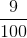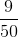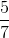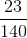Explanation:

First calculate the number of students: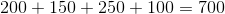The probability of drawing an honors student will then be the total number of honors students divided by the total number of students attending the school: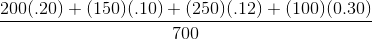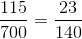### Example Question #62 : Discrete Probability

In a particular high school, 200 students are freshmen, 150 students are sophomores, 250 students are juniors, and 100 students are seniors. Twenty percent of freshmen are in honors classes, ten percent of sophomores are in honors classes, twelve percent of juniors are in honors classes, and thirty percent of seniors are in honors classes.

If a student is chosen at random, what is the probability that that student will be a senior student and a student who does not attend honors classes?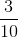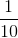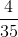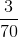Explanation:

First calculate the number of students:The percentage of seniors that do not attend honors classes is: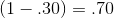Therefore, the probability of selecting a student who is a senior and one who does not attend honors classes is: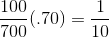### Example Question #101 : How To Find The Probability Of An Outcome

11 cards are placed into a box numbered 5 - 15. If one card is randomly drawn from the box, what is the probabiltiy that a prime number will be on the card?

3/5

6/11

2/5

4/11

1/2

4/11

Explanation:

Possible numbers 5,6,7,8,9,10,11,12,13,14,15 (11 total numbers)

Prime numbers are 5,7,11,13 (4 total prime numbers)

totalnumber of prime numbers/ total numbers in box

### Example Question #1685 : Ssat Upper Level Quantitative (Math)

Aaron, Gary, Craig, and Boone are sitting down in a row of four chairs. What is the probability that Aaron and Gary will be seated beside each other?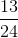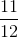Explanation:

Consider first all of the possible ways the men may be arranged, which is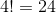Now, consider all of the ways that Aaron and Gary could be seated beside each other; it may be easier to visualize by drawing it out:

1. A G _ _
2. G A _ _
3. _ A G _
4. _ G A _
5. _ _ A G
6. _ _ G A

As seen, there are six possibilities.

Finally, for each of these cases, Craige and Boone could be seated in one of two ways.

So the probability that Aaron and Gary will be seated beside each other is: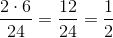1 2 13 14 15 16 17 18 19 21 Next →

### All PSAT Math Resources